Definite Integration - By Substitution

Chapter 7 Class 12 Integrals
Concept wise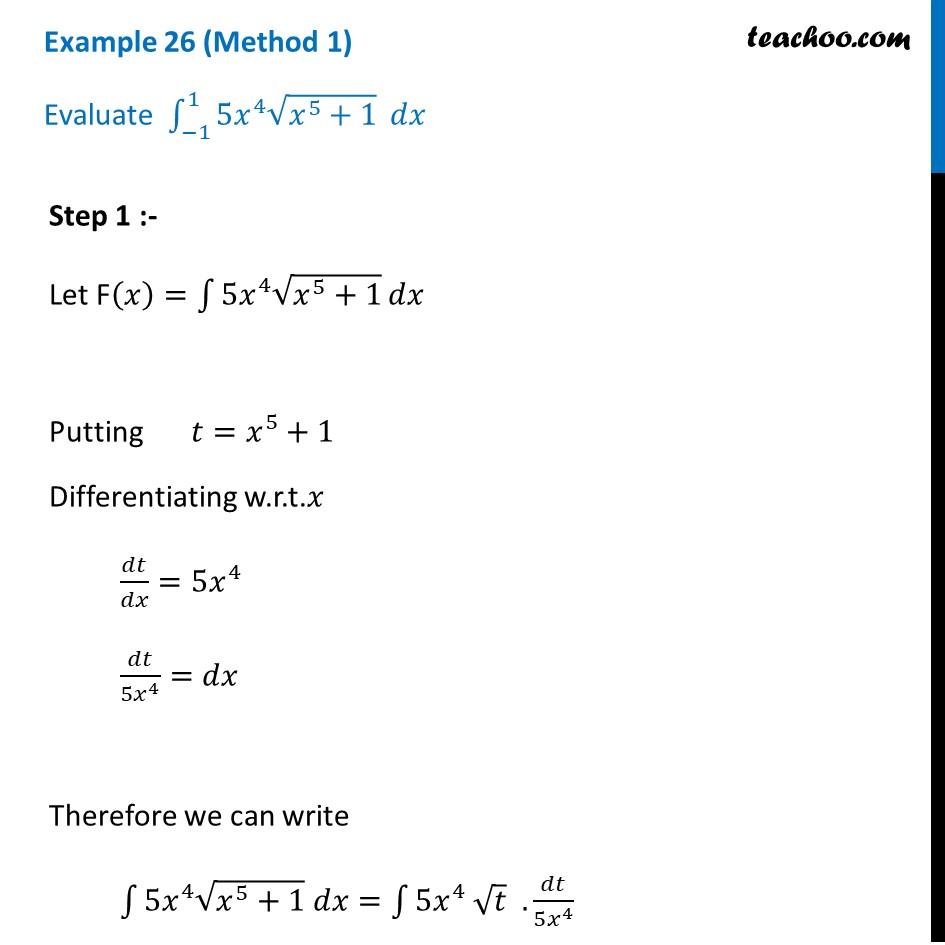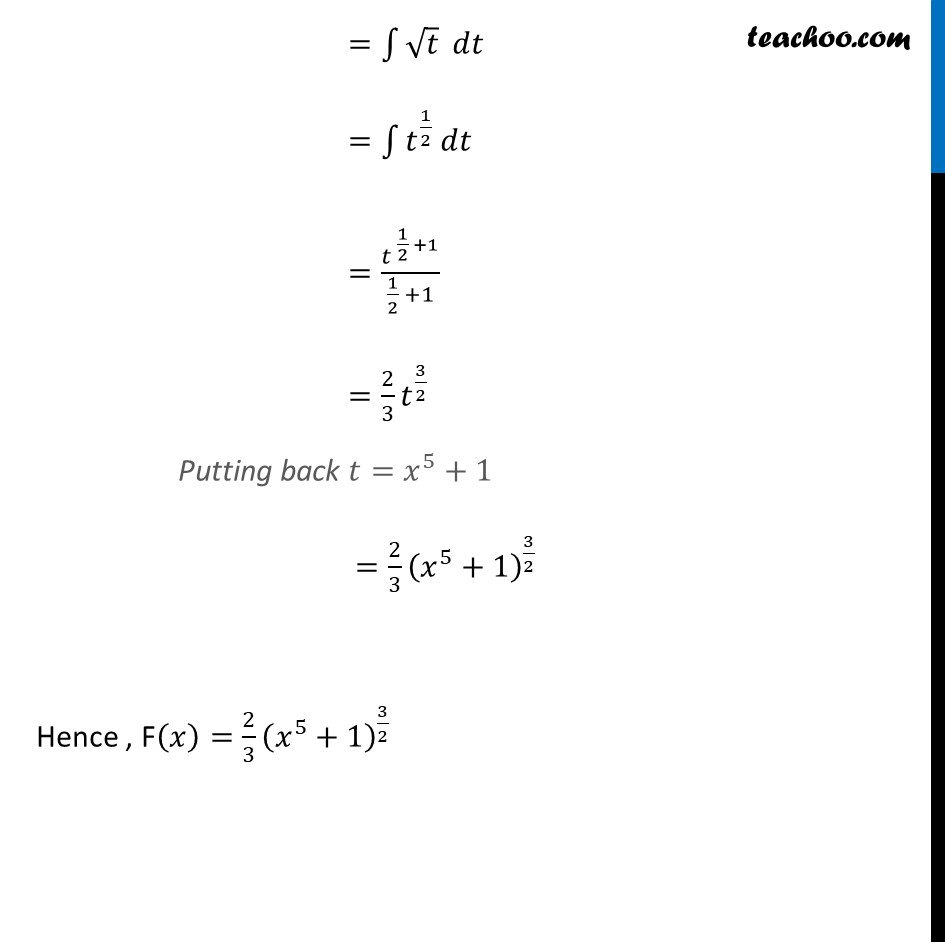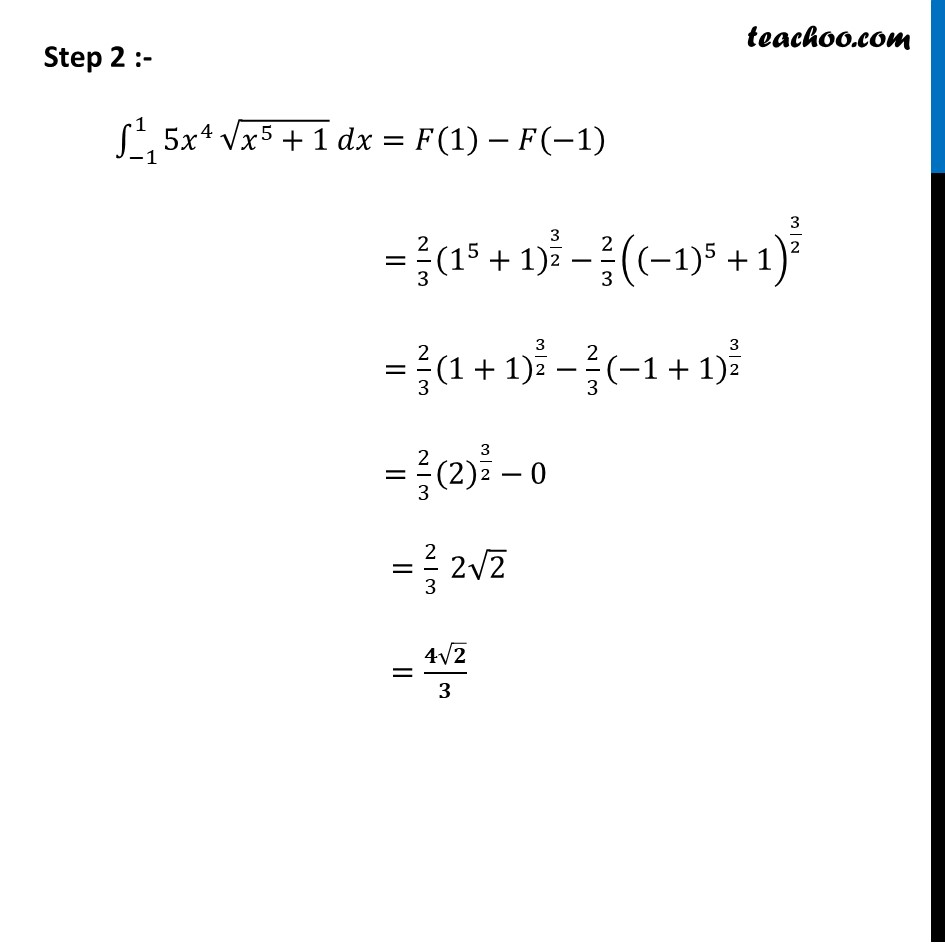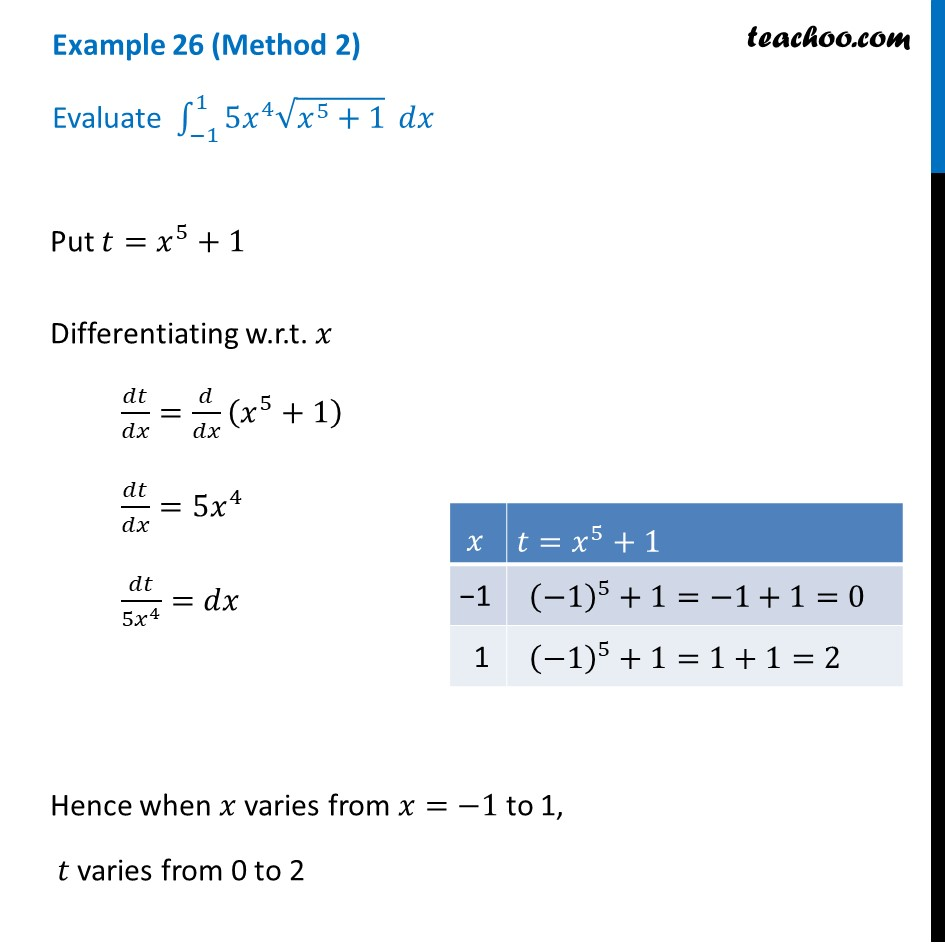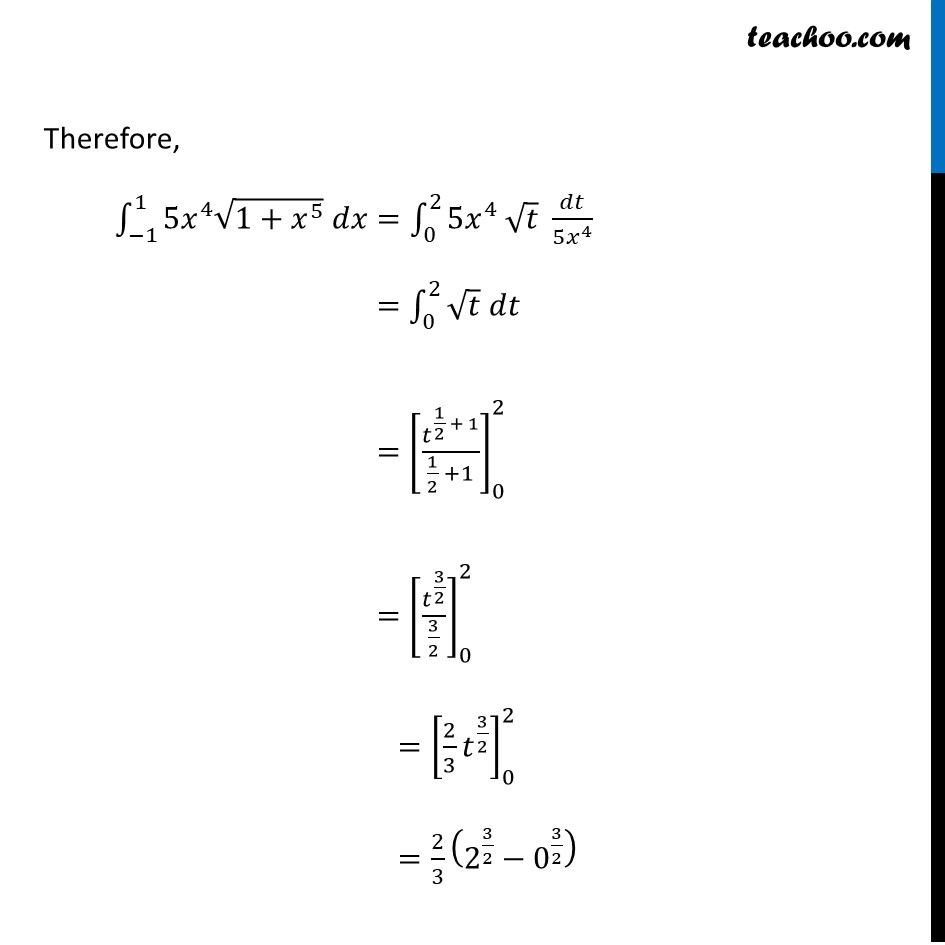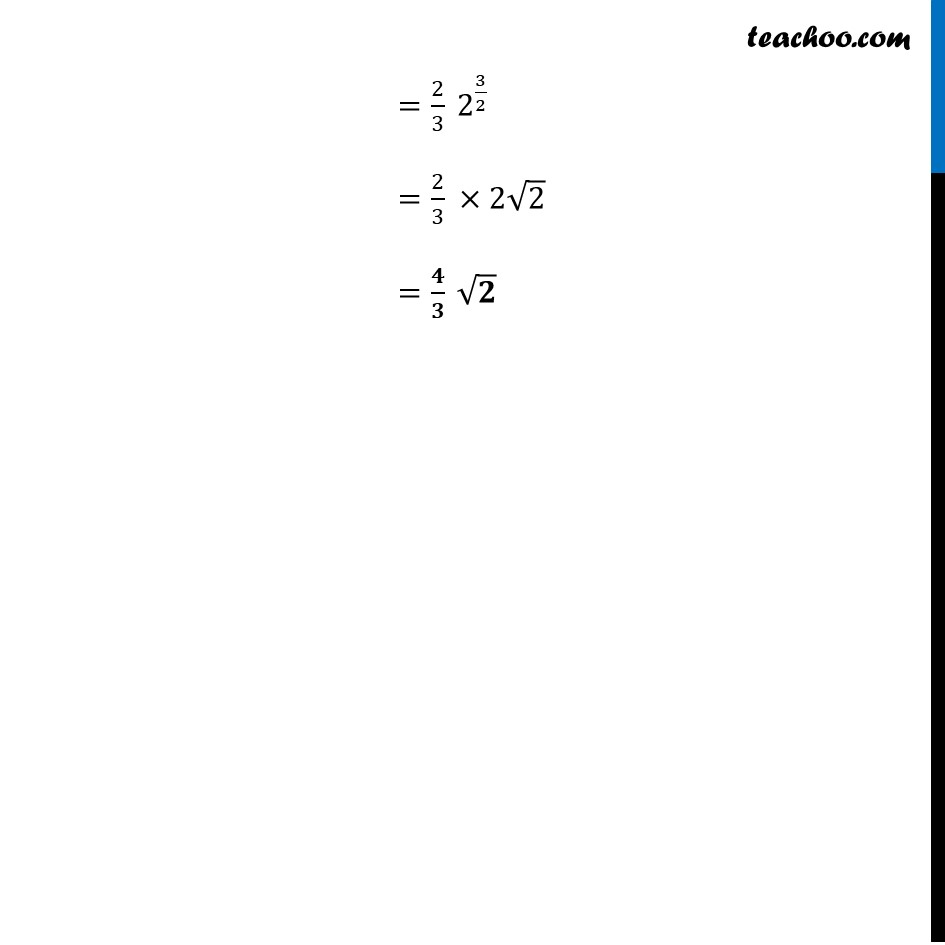Learn in your speed, with individual attention - Teachoo Maths 1-on-1 Class

### Transcript

Example 26 (Method 1) Evaluate ∫_(−1)^1▒〖5𝑥^4 √(𝑥^5+1)〗 𝑑𝑥 Step 1 :- Let F(𝑥)=∫1▒〖5𝑥^4 √(𝑥^5+1)〗 𝑑𝑥 Putting 𝑡=𝑥^5+1 Differentiating w.r.t.𝑥 𝑑𝑡/𝑑𝑥=5𝑥^4 𝑑𝑡/(5𝑥^4 )=𝑑𝑥 Therefore we can write ∫1▒〖5𝑥^4 √(𝑥^5+1) 𝑑𝑥=∫1▒〖5𝑥^4 √𝑡 . 𝑑𝑡/(5𝑥^4 )〗〗 =∫1▒√𝑡 𝑑𝑡 =∫1▒〖𝑡^(1/2) 𝑑𝑡〗 =〖𝑡 〗^(1/2 +1)/(1/2 +1) =2/3 𝑡^(3/2) Putting back 𝑡=𝑥^5+1 =2/3 (𝑥^5+1)^(3/2) Hence , F(𝑥)=2/3 (𝑥^5+1)^(3/2) Step 2 :- ∫_(−1)^1▒〖5𝑥^4 〗 √(𝑥^5+1) 𝑑𝑥=𝐹(1)−𝐹(−1) =2/3 (1^5+1)^(3/2)−2/3 ((−1)^5+1)^(3/2) =2/3 (1+1)^(3/2)−2/3 (−1+1)^(3/2) =2/3 (2)^(3/2)−0 =2/3 2√2 =(𝟒√𝟐)/𝟑 Example 26 (Method 2) Evaluate ∫_(−1)^1▒〖5𝑥^4 √(𝑥^5+1)〗 𝑑𝑥 Put 𝑡=𝑥^5+1 Differentiating w.r.t. 𝑥 𝑑𝑡/𝑑𝑥=𝑑/𝑑𝑥 (𝑥^5+1) 𝑑𝑡/𝑑𝑥=5𝑥^4 𝑑𝑡/(5𝑥^4 )=𝑑𝑥 Hence when 𝑥 varies from 𝑥=−1 to 1, 𝑡 varies from 0 to 2 Therefore, ∫_(−1)^1▒〖5𝑥^4 √(1+𝑥^5 ) 𝑑𝑥=∫_0^2▒〖5𝑥^4 √𝑡 𝑑𝑡/(5𝑥^4 )〗〗 =∫1_0^2▒〖√𝑡 𝑑𝑡〗 =[𝑡^(1/2 + 1)/(1/2 +1)]_0^2 =[𝑡^(3/2)/(3/2)]_0^2 =[2/3 𝑡^(3/2) ]_0^2 =2/3 (2^(3/2)−0^(3/2) ) =2/3 2^(3/2) =2/3 ×2√2 =𝟒/𝟑 √𝟐